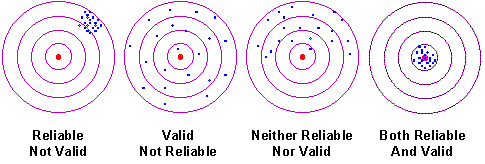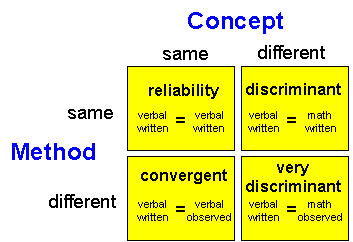Reliability & Validity

# Reliability & Validity

We often think of reliability and validity as separate ideas but, in fact, they’re related to each other. Here, I want to show you two ways you can think about their relationship.

One of my favorite metaphors for the relationship between reliability is that of the target. Think of the center of the target as the concept that you are trying to measure. Imagine that for each person you are measuring, you are taking a shot at the target. If you measure the concept perfectly for a person, you are hitting the center of the target. If you don’t, you are missing the center. The more you are off for that person, the further you are from the center.The figure above shows four possible situations. In the first one, you are hitting the target consistently, but you are missing the center of the target. That is, you are consistently and systematically measuring the wrong value for all respondents. This measure is reliable, but no valid (that is, it’s consistent but wrong). The second, shows hits that are randomly spread across the target. You seldom hit the center of the target but, on average, you are getting the right answer for the group (but not very well for individuals). In this case, you get a valid group estimate, but you are inconsistent. Here, you can clearly see that reliability is directly related to the variability of your measure. The third scenario shows a case where your hits are spread across the target and you are consistently missing the center. Your measure in this case is neither reliable nor valid. Finally, we see the “Robin Hood” scenario – you consistently hit the center of the target. Your measure is both reliable and valid (I bet you never thought of Robin Hood in those terms before).

Another way we can think about the relationship between reliability and validity is shown in the figure below. Here, we set up a 2x2 table. The columns of the table indicate whether you are trying to measure the same or different concepts. The rows show whether you are using the same or different methods of measurement. Imagine that we have two concepts we would like to measure, student verbal and math ability. Furthermore, imagine that we can measure each of these in two ways. First, we can use a written, paper-and-pencil exam (very much like the SAT or GRE exams). Second, we can ask the student’s classroom teacher to give us a rating of the student’s ability based on their own classroom observation.The first cell on the upper left shows the comparison of the verbal written test score with the verbal written test score. But how can we compare the same measure with itself? We could do this by estimating the reliability of the written test through a test-retest correlation, parallel forms, or an internal consistency measure (See Types of Reliability). What we are estimating in this cell is the reliability of the measure.

The cell on the lower left shows a comparison of the verbal written measure with the verbal teacher observation rating. Because we are trying to measure the same concept, we are looking at convergent validity (See Measurement Validity Types).

The cell on the upper right shows the comparison of the verbal written exam with the math written exam. Here, we are comparing two different concepts (verbal versus math) and so we would expect the relationship to be lower than a comparison of the same concept with itself (e.g., verbal versus verbal or math versus math). Thus, we are trying to discriminate between two concepts and we would consider this discriminant validity.

Finally, we have the cell on the lower right. Here, we are comparing the verbal written exam with the math teacher observation rating. Like the cell on the upper right, we are also trying to compare two different concepts (verbal versus math) and so this is a discriminant validity estimate. But here, we are also trying to compare two different methods of measurement (written exam versus teacher observation rating). So, we’ll call this very discriminant to indicate that we would expect the relationship in this cell to be even lower than in the one above it.

The four cells incorporate the different values that we examine in the multitrait-multimethod approach to estimating construct validity.

When we look at reliability and validity in this way, we see that, rather than being distinct, they actually form a continuum. On one end is the situation where the concepts and methods of measurement are the same (reliability) and on the other is the situation where concepts and methods of measurement are different (very discriminant validity).